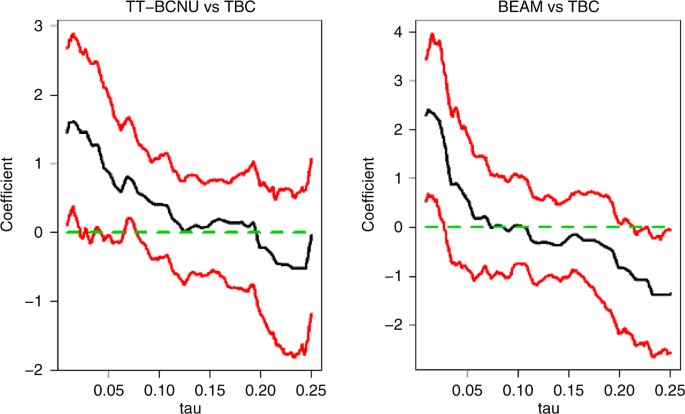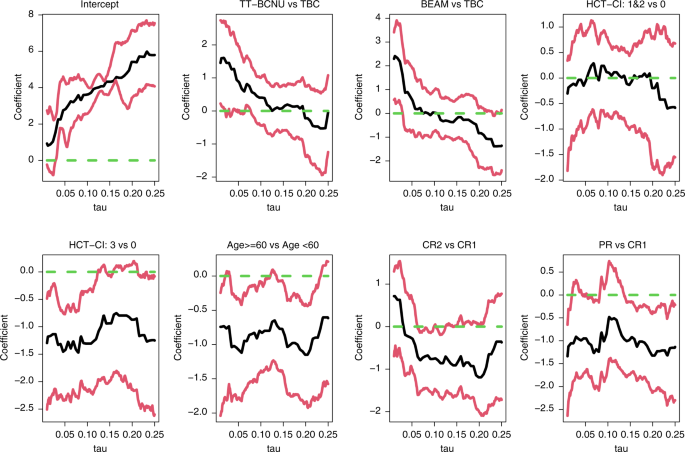## Introduction

An important problem in haematopoietic cell transplant research is to assess the relationship between survival time and/or time to relapse and exploratory co-variates such as age, sex and therapy. In most studies survival outcomes are censored because of incomplete follow-up, withdrawal of consent and other reasons. The most common way to analyze censored data in transplant studies is the Cox proportional hazards model where the key idea is to evaluate the effect of a co-variate on the hazard rate (instantaneous rate of failure). This approach lacks direct physical interpretation and may be unattractive to some physicians and statisticians . The accelerated failure time (AFT) model which directly evaluates the association between the survival outcome and co-variates is another common way to deal with censored data. However, the Cox proportional hazards and AFT models are problematic because of strong assumptions such as proportional hazards and homogenous effect, assumptions which often do not operate in real data. For example, analyses of a Center for International Blood and Marrow Transplant Research (CIBMTR) registry study of transplants in primary central nervous system lymphoma (PCNSL) reported survival data violated the proportional hazard assumption of the Cox model . Also, these models also confer only a static view of the treatment effect.

Quantile regression has become a popular alternative to the Cox proportional hazards and AFT models in survival analyses [3,4,5,6,7,8,9]. Quantile regression has these advantages:  relaxes the proportional hazards and homogenous effect assumptions and allows for heterogeneous co-variates effects;  is a robust quantity tool to outliers and censoring because quantiles of survival time are more identifiable compared with mean survival time when there is censoring with bounded support;  provides a straightforward physical interpretation; and  has flexibility in exploring the dynamic relationship between survival and co-variates of interest. These features of quantile regression guarantee its usefulness in exploring and identifying heterogenous co-variate effects in censored data. A comprehensive overview of quantile regression approach is available .

In this typescript I concisely introduce the quantile regression model for right censored data. I use data from the aforementioned PCNSL study to illustrate how to use the quantile regression and interpret the results. Lastly, briefly discuss use of quantile regression in complex survival analyses such as competing risk data or non-compliance data.

## Censored quantile regression

First, I introduce the concepts of quantile and of censored quantile regression. For any τ between 0 and 1, the τ-th quantile can be intuitively explained as a cut-off point where τ fraction of the data are at or below. Quantile at some specific τ’s is already commonly used in biomedical studies. For example, the 0.5-th quantile of survival time referred to as median survival time, the most commonly reported survival outcome. Rigorously, the τ-th quantile of a random variable Y, denoted by QY(τ), is defined as inf{y:Pr(Y ≤ y) ≥ τ}. If there is a co-variate X, the τ-th conditional quantile of Y given X, QY(τ|X), is defined by inf{y:Pr(Y ≤ y|X) ≥ τ}. Suppose T and X denote the survival time and sex (1: male; 0: female), QT(0.5|X = 1) represents the median survival time in males.

For the survival time T and co-variates X, the standard censored quantile regression model assumes the τ-th conditional quantile of log(T) given X is a linear combination of X, which is formulated as

$$Q_{\log \left( T \right)}\left( {\tau |X} \right) = {{{{{{{\boldsymbol{X}}}}}}}}^ \top {\it{\upbeta }}\left( \tau \right),\tau \in (0,1)$$
(1)

where β(τ) represents the effects of covariate X on the τ-th conditional quantile of log(T). Model (1) permits the quantile-varying co-variate effects by allowing β(τ) to change with τ. This feature of the quantile regression provides the flexibility to accommodate heterogenous co-variate effects.

Let C denote the potential censoring time in right censored data. We can only have Y = min(T,C) and δ = 1(T ≤ C), the observed survival time and the non-censoring indicator instead of T in the data. Several approaches have been developed to tackle censoring under the conditionally random right censoring assumption which assumes C is independent of T given co-variates X. For example, Portnoy proposed a recursive re-weighting algorithm by adopting the principle of self-consistency for the Kaplan-Meier estimator [7, 11]. Peng and Huang derived a stochastic integral based estimating equation of model (1) by utilizing the martingale structure underlying randomly censored data . Both methods have been implemented in crq() function in the R package quantreg  and PROC QUANTLIFE in SAS.

## An example

Next, I consider a PCNSL study by CIBMTR published in 2021 . The study included 603 subjects with PCNSL receiving an autotransplant, 263 (44%) of whom received thiotepa/busulfan/cyclophosphamide (TBC) for pretransplant conditioning, 275 (45%), thiotepa/carmustine (TT-BCNU) and 65 (11%), carmustine/etoposide/cytarabine/melphalan (BEAM). The study objective was to interrogate associations between conditioning regimen and survival. In the analysis the authors determined the proportional hazard assumption is violated and constructed a piecewise proportional hazards model with a cutoff at 6 months . With this approach the data indicated use of TT-BCNU (HR = 0.35; 95% Confidence Interval [CI], [0.17, 0.37]; P = 0.01) was associated with a lower risk of death in ≤ 6 months compared with the TBC regimen and with a higher risk of death after 6 months (HR = 1.54 [0.93, 2.55]; P = 0.10). The BEAM regimen was associated with a lower risk of death in first 6 month (HR = 0.26 [0.06, 1.12]; P = 0.07), and with a higher risk of death at > 6 months (HR = 2.73 [1.56, 4.76]; P < 0.001) compared with the TBC regimen. Importantly, it is difficult for biomedical researchers and physicians to directly interpret these time-varying effects.

To address the violation of proportional hazards assumption with quantile regression model I applied a censored quantile regression model to interrogate the relationship between the three regimens and survival adjusting for the risk factors including age, hematopoietic cell transplant comorbidity index (HCT-CI) and disease state. Because the percentage of deaths is around 20%, I set τU = 0.25 to avoid an unstable estimator at high quantiles. Using the crq() function in R package quantreg the estimated coefficients with its 95% point-wise confidence intervals of TT-BCNU and BEAM compared with TBC are displayed in Fig. 1. The estimated coefficients of other co-variates are displayed in Fig. 2. Fig. 1 shows the estimated coefficient of TT-BCNU compared with TBC decreases as τ increases. For example, Fig. 2 shows the intercept coefficient estimate at τ = 0.10 equals 3.62 ([2.56, 4.09]; P < 0.001) indicating the 10th quantile of survival time in reference subjects (age < 60 years, HCT-CI = 0 in 1st complete remission receiving TBC) is 37.5 (=exp[3.62], [12.93, 59.60]; P < 0.001) months. The corresponding estimated coefficient of TT-BCNU at τ = 0.10 equals 0.40 ([−0.43, 1.00]; P = 0.27) suggesting subjects receiving TT-BCNU live 1.5 months(=exp[0.40], [0.65, 2.73]; P = 0.27) longer than those not receiving it at 10th quantile. The estimated coefficient of TT-BCNU at τ = 0.15, 0.11 ([−0.60, 0.79]; P = 0.75) suggests receiving TT-BCNU prolongs 15th quantile survival by about 1 month(=exp[0.11]; [0.55, 2.20]; P = 0.75). The estimated coefficient of TT-BCNU is only significantly above 0 when τ < 0.03, suggesting TT-BCNU significantly prolong survival only for subjects with low quantiles of survival (e.g., the worst subjects) and the survival benefit of TT-BCNU decreases for subjects with high quantiles of survival (e.g., better subjects). The estimated coefficient of BEAM decreases as τ increases and is below 0 with τ ≥ 0.11. The estimated coefficient of BEAM at τ = 0.15, −0.22 ([−1.05, 0.59]; P = 0.60), suggests the 15th quantile of survival time of subjects receiving BEAM is only 0.8 (=exp[−0.22]; [0.35 ‒1.80]; P = 0.60) times compared to the 15th quantile of survival time of reference subjects. Moreover, the estimated coefficient of BEAM is significantly below 0 when τ ≥ 0.22 suggesting BEAM may be harmful to better subjects. Note all these interpretations and results are point-wise. If researchers are interested in assessing whether the average effect among a region of τ is above 0 they can use the second stage inference procedure described in . These results confirm conclusions in the CIBMTR article that TT-BCNU is only associated with lower risk of death in ≤ 6 months whereas BEAM is only associated with a higher risk of death at > 6 months. Moreover, compared with results from the piecewise Cox proportional hazards model, results of the quantile regression model displayed in Figs. 1 and 2 indicate co-variate effects and each quantile and give detailed insights into the relationship between survival and the co-variates.Fig. 1: The results on the estimated coefficients of TT-BCNU and BEAM from censored quantile regression model.Fig. 2: Results on estimated coefficients of all co-variates from censored quantile regression model.

## Discussion

Quantile regression is a powerful approach for analyzing censored data with heterogenous co-variate effects with advantages over other methods of survival analyses in depicting the dynamic association between survival outcome and co-variates. I show how censored quantile regression operates by re-analyzing CIBMTR data for transplants in PCNSL. Results from censored quantile regression can verify results of the piecewise Cox proportional hazards model and give insights about co-variates effect the survival distribution. Unlike the selected ad-hoc cutoffs in piecewise Cox proportional hazards model the quantile regression provides a natural cutoff, quantile to describe heterogenous effects. I recommend researchers consider the censored quantile regression over the Cox proportional hazards or AFT models when they analyze censored data with heterogenous co-variates effects.

More recent developments of censored quantile regression such as censored quantile regression for competing and semi-competing risk data, truncated data, recurrent event data and censored quantile regression in a causal framework are also worth considering and can be easily implemented in existing statistical software, such as R or SAS [12,13,14,15,16].